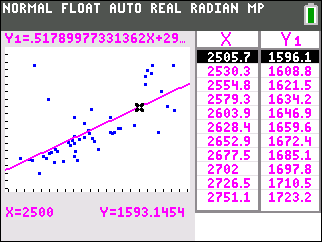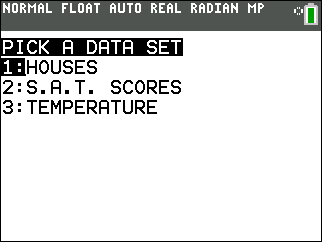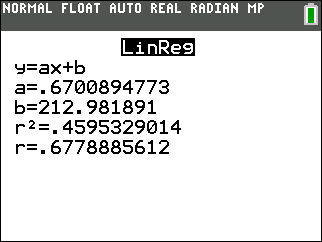••• ##### Device
• TI-83 Plus Family
• TI-84 Plus
• TI-84 Plus Silver Edition
•TI-84 Plus C Silver Edition
•TI-84 Plus CE

# Statistics: Does a Correlation Exist?

by Texas Instruments#### Overview

Students determine, by examining a graph, if a data set has a positive or negative correlation coefficient.

#### Key Steps

•Students explore correlation coefficients and linear regression lines. Each problem contains a different data set and each problem has students investigate something different. They will create a scatter plot and use the calculator to find the equation of the regression.

•Students use the regression line to find and predict an x-value when given a y-value and vice versa. They will also determine the difference between correlation and causation.

•Lastly, students will explore the effect of extreme data point on the regression line. After changing a data point, they will update the linear regression line and equation to see the effect. They will also delete data points.